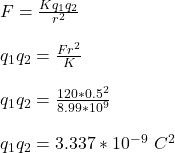Question

Two small conducting point charges, separated by 0.5 m, carry a total charge of 170 mu or micro CC. They repel one another with a force of 120 N. For the universal constant k use the value 8.99 times 109 N m2/C2.
Find the charge on the larger of the two point charges.

1.the two point charges are equal

q₁ = 147.353 x 10⁻⁶ C or 22.646 x 10⁻⁶ C

q₂ =  22.647 x 10⁻⁶ C  or 147.354 x 10⁻⁶ C

Explanation:

Given;

distance between the two charges, r =  0.5 m

let the first charge = q₁

let the second charge = q₂

q₁ + q₂ = 170μ = 170 x 10⁻⁶ C ———- equation (1)

Force of repulsion between the two charges, q₁ and q₂ = 120 N

Coulomb’s constant, K =  8.99 x 10⁹ N m²/C²

Apply coulomb’s law, for force of attraction or repulsion between two point charges;————–  equation (2)

From equation (1); q₂ = 170 x 10⁻⁶ C  – q₁

Substitute in the value of q₂ in equation (2)

q₁(170 x 10⁻⁶  – q₁) = 3.337 x 10⁻⁹

170 x 10⁻⁶q₁ – q₁² = 3.337 x 10⁻⁹

170 x 10⁻⁶q₁ = q₁² + 3.337 x 10⁻⁹

0 = q₁² – 170 x 10⁻⁶q₁ + 3.337 x 10⁻⁹ (this is quadratic equation)

q₁ = 147.353 x 10⁻⁶ C or 22.646 x 10⁻⁶ C

q₂ = 170 x 10⁻⁶ C  – q₁

q₂ = 170 x 10⁻⁶ C – 147.353 x 10⁻⁶ C     or    170 x 10⁻⁶ C –  22.646 x 10⁻⁶ C

q₂ =  22.647 x 10⁻⁶ C  or 147.354 x 10⁻⁶ C

Therefore, the two point charges are equal